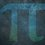# UKMT Special (Problem $2$)

The largest of $4$ different real numbers is $d$.

When the numbers are summed in pairs, the four largest sums are

$9, 10, 12, 13$

What are the possible values of $d$?

[UKMT Hamilton Olympiad $2017$, H$4$]Note by Yajat Shamji
8 months, 2 weeks ago

This discussion board is a place to discuss our Daily Challenges and the math and science related to those challenges. Explanations are more than just a solution — they should explain the steps and thinking strategies that you used to obtain the solution. Comments should further the discussion of math and science.

When posting on Brilliant:

• Use the emojis to react to an explanation, whether you're congratulating a job well done , or just really confused .
• Ask specific questions about the challenge or the steps in somebody's explanation. Well-posed questions can add a lot to the discussion, but posting "I don't understand!" doesn't help anyone.
• Try to contribute something new to the discussion, whether it is an extension, generalization or other idea related to the challenge.

MarkdownAppears as
*italics* or _italics_ italics
**bold** or __bold__ bold
- bulleted- list
• bulleted
• list
1. numbered2. list
1. numbered
2. list
Note: you must add a full line of space before and after lists for them to show up correctly
paragraph 1paragraph 2

paragraph 1

paragraph 2

[example link](https://brilliant.org)example link
> This is a quote
This is a quote
    # I indented these lines
# 4 spaces, and now they show
# up as a code block.

print "hello world"
# I indented these lines
# 4 spaces, and now they show
# up as a code block.

print "hello world"
MathAppears as
Remember to wrap math in $$ ... $$ or $ ... $ to ensure proper formatting.
2 \times 3 $2 \times 3$
2^{34} $2^{34}$
a_{i-1} $a_{i-1}$
\frac{2}{3} $\frac{2}{3}$
\sqrt{2} $\sqrt{2}$
\sum_{i=1}^3 $\sum_{i=1}^3$
\sin \theta $\sin \theta$
\boxed{123} $\boxed{123}$

Sort by:

You have until next Sunday, $3:00$pm!

- 8 months, 2 weeks ago

Let the other $3$ numbers be $a, b, c$ in increasing order. Then we know that $c+d$ is the largest sum, and $b+d$ is the next largest. Whether $a+d$ or $b+c$ is greater cannot be guaranteed. Let us do this in two cases -

Case $1$ - $a+d$ is larger than $b+c$

Then, we know that $b+c$ must be fourth largest. Thus, we have the following equations,

$c + d = 13 \ldots \text{eq(1)} \\ b + d = 12 \ldots \text{eq(2)} \\ a+d = 10 \ldots \text{eq(3)} \\ b + c = 9 \ldots \text{eq(4)}$

From equations $1$ and $4$, we can simplify to get $b = d - 4 \ldots \text{eq(5)}$

From equations $2$ and $5$ now, we can simplify to get $\to d = 8$ (and $b=4$, which can help us solve for $a$ and $c$, but that is not required)

First Case : $d = 8$

Case $2$ - $b+c$ is larger than $a+d$

Then, we know that $b+c$ must be fourth largest. Thus, we have the following equations,

$c + d = 13 \ldots \text{eq(1)} \\ b + d = 12 \ldots \text{eq(2)} \\ b+c = 10 \ldots \text{eq(3)} \\ a+d = 9 \ldots \text{eq(4)}$

From equations $2$ and $3$, we can simplify to get $c = d - 2 \ldots \text{eq(5)}$

From equations $1$ and $5$ now, we can simplify to get $\to d = 7.5$ (and $c=5.5$, which can help us solve for $a$ and $b$, but again, that is not required)

Second Case : $d = 7.5$

Thus,

$d = 8 \text{ or } 7.5$

- 8 months, 2 weeks ago

@Yajat Shamji - Where did you find these problems? They are interestingly easy lol

- 8 months, 2 weeks ago

Go to this note:

- 8 months, 2 weeks ago

Nice :)

- 8 months, 2 weeks ago

But... slightly different method.

- 8 months, 2 weeks ago

Ok (some text)

- 8 months, 2 weeks ago